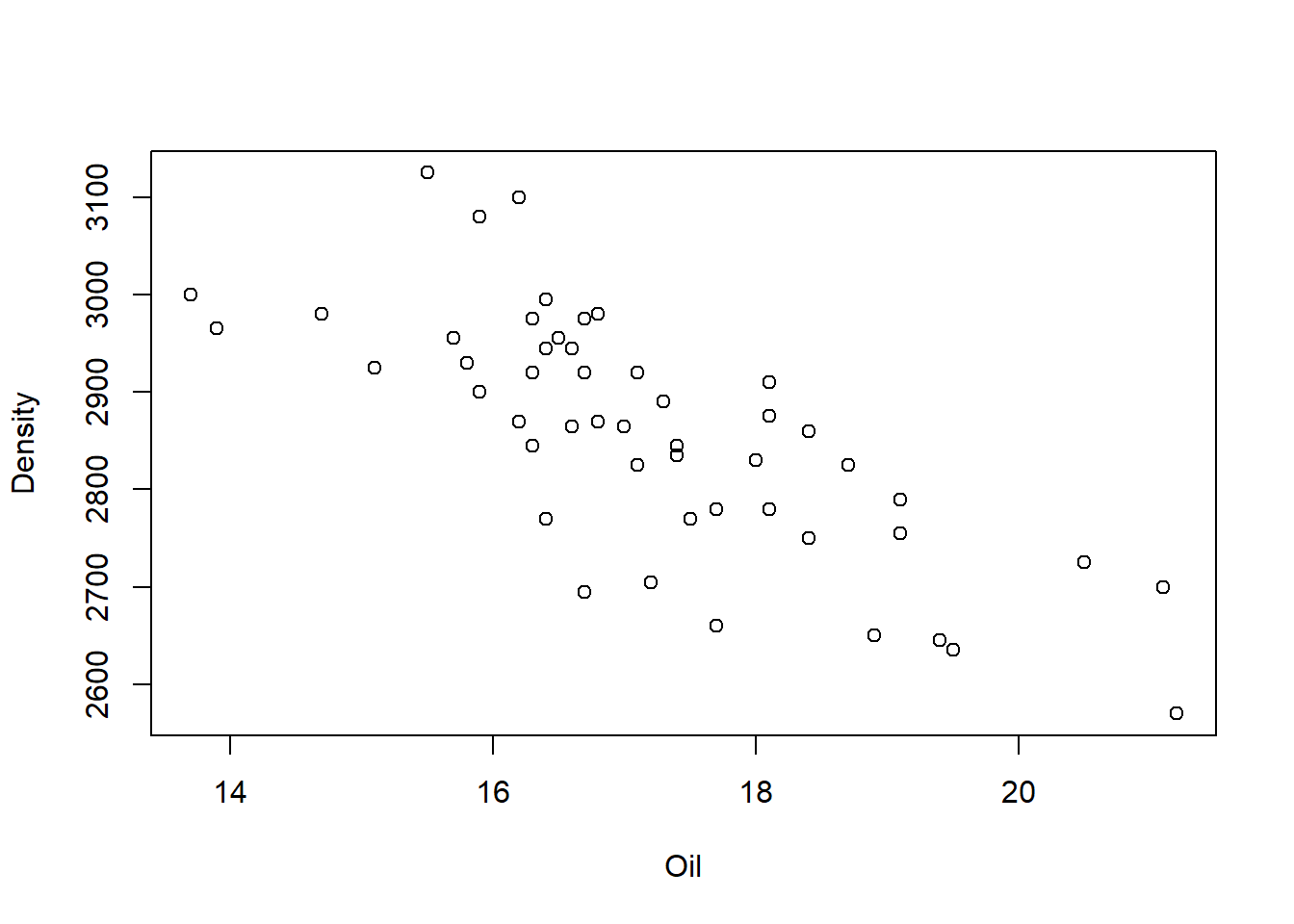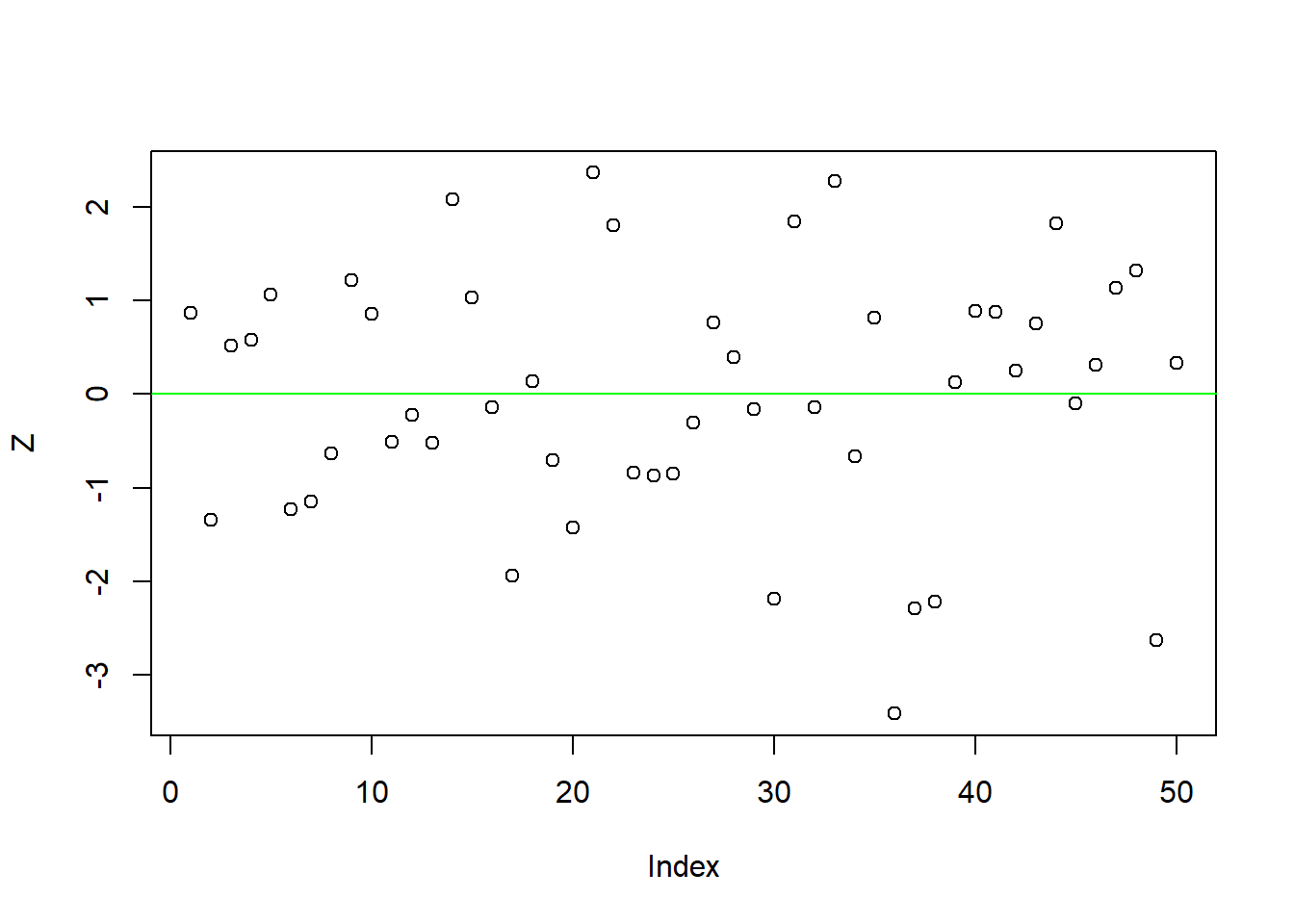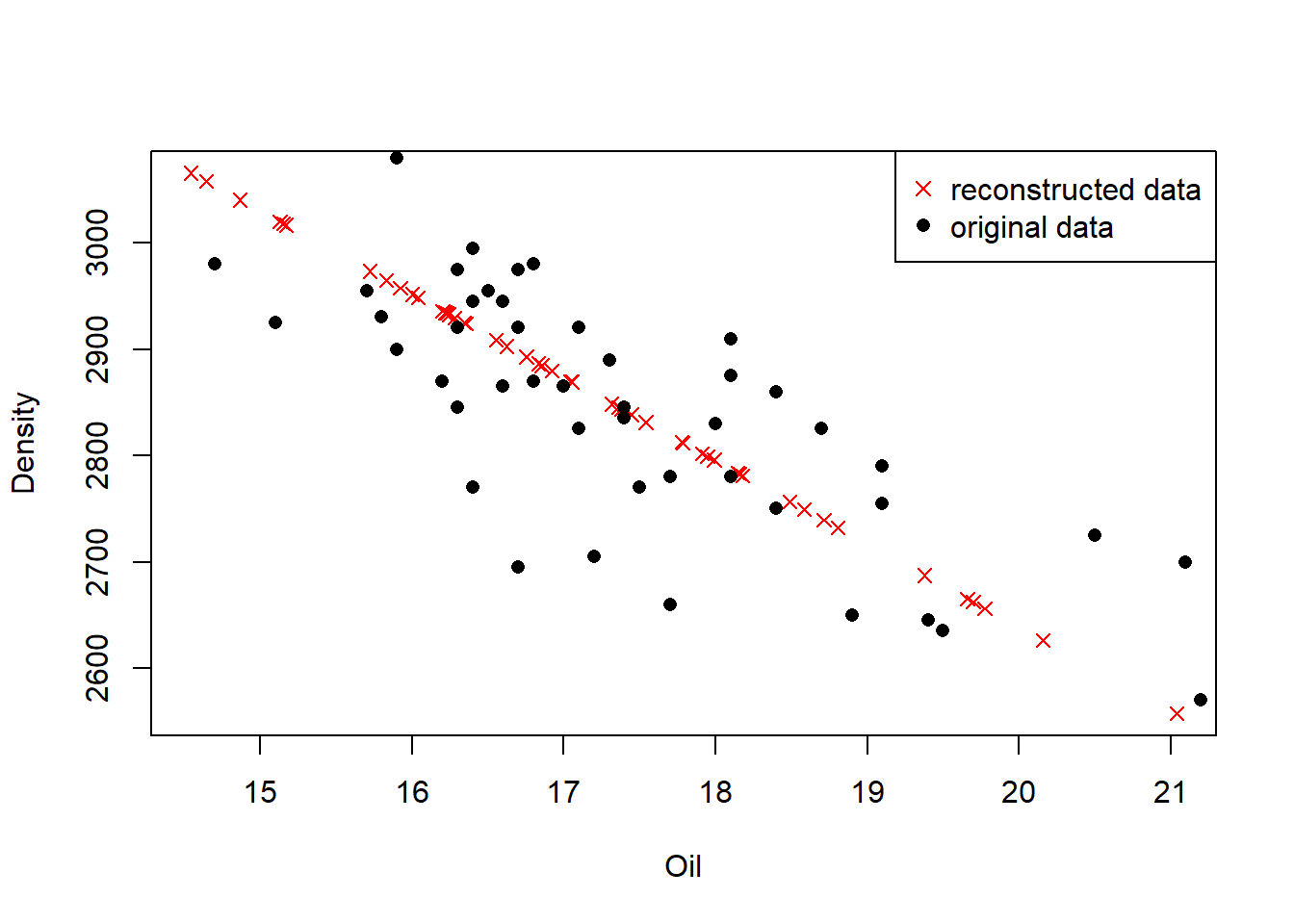In this section we show how to restore the original data. We showcase the procedure on the simplified food-texture data set. First we load the data, extract the two featuresOil and Density, and thereafter we plot the data.

food <- read.csv("food-texture.csv")
pca_toy <- food[, c("Oil", "Density")]
plot(pca_toy)The data set contains 50 rows (observations) and 2 columns (features).

In the next step we center and scale the data, and calculate the eigenvalue of the data covariance matrix to extract the principal components loading vector. To reduce the amount of coding we make use of the pipe operator %>% provided by the dplyr package.

# load helper functions from previous sections

library(dplyr)
# center and scale data set
pca_toy_data <- pca_toy %>%
apply(MARGIN = 2, FUN = center) %>%
apply(MARGIN = 2, FUN = scale)

pca_toy_loading
##            [,1]       [,2]
## [1,] -0.7071068 -0.7071068
## [2,]  0.7071068 -0.7071068

Then, for the sake simplicity we pick only the first principal component and project the original two-dimensional data onto an one-dimensional feature space by multiplying the data matrix with the loading vector.

$\mathbf{Z}_{n×k}=\mathbf{X}_{n×d}\mathbf{P}_{d×k}$

P <- pca_toy_loading[, 1]
Z <- pca_toy_data %*% P
plot(Z)
abline(h = 0, col = "green")Done! We projected our original data set onto an one-dimensional feature space.

Before we get the original data back, remember that only if we took all the eigenvectors in our transformation will we get exactly the original data back. As we reduced the number of eigenvectors in the final transformation, the retrieved data has lost some information.

In order to re-project the data onto the original two-dimensional feature space we rearrange the equation from above

$\mathbf{Z}=\mathbf{XP}⇒\mathbf{X}=\mathbf{ZP^{-1}}⇒\mathbf{X}=\mathbf{ZP^{T}}$

The inverse of the feature vector ($$\mathbf{P}^{−1}$$) is equal to the transpose of the feature vector ($$\mathbf{P}^{T}$$), because the elements of the matrix are all the unit eigenvectors of the data set and thus are orthonormal.

X <- Z %*% t(P)
dim(X)
##  50  2

Perfect, our data is re-projected to 50 rows (observations) and 2 columns (features). Before we plot the result and compare it to the raw data, we first have to invert the standardization procedure, hence we have to multiply the reconstructed data with the standard deviation and add the mean.

X <- Z %*% t(P)
pca_toy_mean <- apply(pca_toy, 2, mean) # calculate mean
pca_toy_sd <- apply(pca_toy, 2, sd) # calculate standard deviation

# invert the standardization procedure
X[, 1] <- X[, 1] * pca_toy_sd + pca_toy_mean
X[, 2] <- X[, 2] * pca_toy_sd + pca_toy_mean

plot(X, pch = 4, col = "red", ylab = "Density", xlab = "Oil") # plot reconstructed data
points(pca_toy[, 1], pca_toy[, 2], pch = 16) # plot original data
legend("topright",
legend = c("reconstructed data", "original data"),
pch = c(4, 16),
col = c("red", "black"))Citation

The E-Learning project SOGA-R was developed at the Department of Earth Sciences by Kai Hartmann, Joachim Krois and Annette Rudolph. You can reach us via mail by soga[at]zedat.fu-berlin.de.You may use this project freely under the Creative Commons Attribution-ShareAlike 4.0 International License.

Please cite as follow: Hartmann, K., Krois, J., Rudolph, A. (2023): Statistics and Geodata Analysis using R (SOGA-R). Department of Earth Sciences, Freie Universitaet Berlin.